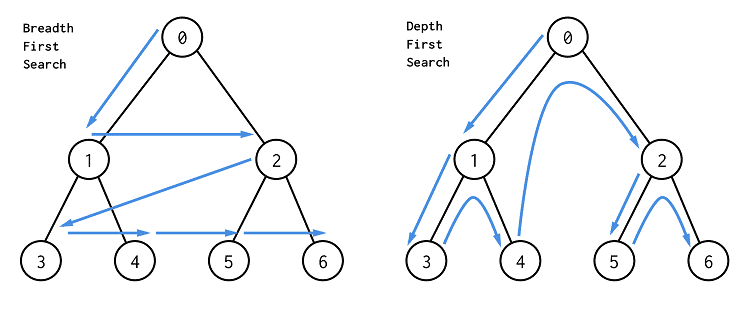# 113. Path Sum II 路径总和 II

@[toc]

## # 题目描述

Given a binary tree and a sum, find all root-to-leaf paths where each path's sum equals the given sum.

Note: A leaf is a node with no children.

Example:

Given the below binary tree and sum = 22,

``````      5
/ \
4   8
/   / \
11  13  4
/  \    / \
7    2  5   1
``````

Return:

``````[
[5,4,11,2],
[5,8,4,5]
]
``````

## # 解题方法### # BFS

BFS使用队列，把每个还没有搜索到的点依次放入队列，然后再弹出队列的头部元素当做当前遍历点。BFS总共有两个模板：

1. 如果不需要确定当前遍历到了哪一层，BFS模板如下。
``````while queue 不空：
cur = queue.pop()
if cur 有效且未被访问过：
进行处理
for 节点 in cur 的所有相邻节点：
if 该节点有效：
queue.push(该节点)
``````
1. 如果要确定当前遍历到了哪一层，BFS模板如下。 这里增加了level表示当前遍历到二叉树中的哪一层了，也可以理解为在一个图中，现在已经走了多少步了。size表示在当前遍历层有多少个元素，也就是队列中的元素数，我们把这些元素一次性遍历完，即把当前层的所有元素都向外走了一步。
``````level = 0
while queue 不空：
size = queue.size()
while (size --) {
cur = queue.pop()
if cur 有效且未被访问过：
进行处理
for 节点 in cur的所有相邻节点：
if 该节点有效：
queue.push(该节点)
}
level ++;
``````

``````# Definition for a binary tree node.
# class TreeNode:
#     def __init__(self, x):
#         self.val = x
#         self.left = None
#         self.right = None

class Solution:
def pathSum(self, root: TreeNode, sum: int) -> List[List[int]]:
res = []
que = deque()
que.append((root, [], 0)) # 将要处理的节点，路径，路径和
while que:
node, path, pathSum = que.popleft()
if not node: # 如果是空节点，不处理
continue
if not node.left and not node.right: # 如果是叶子节点
if node.val + pathSum == sum: # 加上叶子节点后，路径和等于sum
res.append(path + [node.val]) # 保存路径
# 处理左子树
que.append((node.left, path + [node.val], pathSum + node.val))
# 处理右子树
que.append((node.right, path + [node.val], pathSum + node.val))
return res
``````

### # DFS

``````# Definition for a binary tree node.
# class TreeNode:
#     def __init__(self, x):
#         self.val = x
#         self.left = None
#         self.right = None

class Solution:
def pathSum(self, root: TreeNode, sum: int) -> List[List[int]]:
res = []
self.dfs(root, sum, res, [])
return res

def dfs(self, root, sum, res, path):
if not root: # 空节点，不做处理
return
if not root.left and not root.right: # 叶子节点
if sum == root.val: # 剩余的「路径和」恰好等于叶子节点值
res.append(path + [root.val]) # 把该路径放入结果中
self.dfs(root.left, sum - root.val, res, path + [root.val]) # 左子树
self.dfs(root.right, sum - root.val, res, path + [root.val]) # 右子树
``````

## # 日期

2018 年 6 月 22 日 ———— 这周的糟心事终于完了